## Example Questions

2 Next →

### Example Question #11 : How To Find The Volume Of A Cylinder

Find the volume of a cylinder with height 1 and radius 1.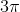Explanation:

To solve, simply use the formula for volume of a cylinder.

First, identify what is known.

Height = 1

Substitute these values into the formula and solve.

Thus,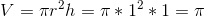### Example Question #12 : How To Find The Volume Of A Cylinder

Find the volume of a cylinder given height ofand radius of.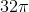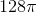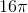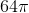Explanation:

To solve, simply use the following formula. Thus,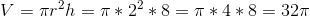### Example Question #13 : How To Find The Volume Of A Cylinder

A cylindrical tank is used as part of a water purifying plant. When contaminated water flows into the top section of the tank, pressure forces it through a mesh filter at the bottom of the tank and clean water exits through a funnel, leaving sediment behind. The tank's filter must be replaced when the total sediment content of the tank exceeds ten percent of the tank's total volume. If the tank is 100 feet tall and 18 feet in diameter, how much sediment, in cubic feet, can the drum hold before the filter must be changed?Explanation:

The volume of a cylinder is found using the following formula:In this formula, the variableis the height of the cylinder andis its radius. Since the diameter is two times the radius, first solve for the radius.Divide both sides of the equation by 2.The given cylinder has a radius of 9 feet. Now, substitute the calculated and known values into the equation for the volume of a cylinder and solve.This is the total volume of the tank. The question asks for the volume of ten percent of the tank—the point at which the filter must be replaced. To find this, move the decimal point in the numerical measure of total volume to the left one place in order to calculate ten percent of the total volume. (Ignore—you can treat it like a multiplier here. Since it appears on both sides of the equals sign, it doesn't affect the decimal shift.)The tank can holdcubic feet of sediment before the filter needs to be changed.

2 Next →